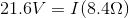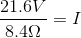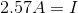# High School Physics : Understanding Current in a Circuit

## Example Questions

### Example Question #29 : Electric Circuits

A closed electric circuit is set up so that there is a resistance of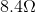and a voltage of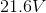. What is the current in the circuit?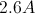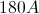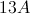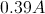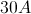Explanation:

For this problem, use Ohm's law: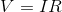.

We are given the voltage and the resistance. Using these terms, we can solve for the current.